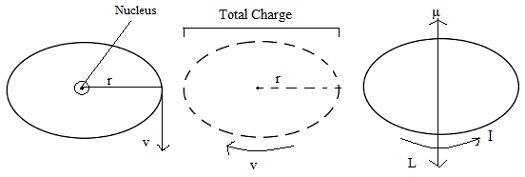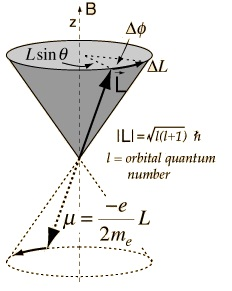#### Magnetism of Materials, Physics tutorial

Introduction to Magnetism of Materials:

Materials like needles, nails, and so on, which are affected by a magnet is termed as magnetic materials while other materials, such as wood or paper, are termed as non-magnetic materials. Though, this doesn't mean that there is no consequence of magnetic field on non-magnetic materials. The difference between the behavior of such materials and iron such as magnetic materials is that the effect of magnetic field on non-magnetic material is extremely weak.

There are two kinds of non-magnetic materials: diamagnetic and paramagnetic.

Response of different substances to a Magnetic Field:

To illustrate how the magnetic materials respond to a magnetic field, consider a strong electromagnet, which consists of one sharply pointed pole piece and one flat pole piece.

The magnetic field is much stronger in the area close to the pointed pole while close to the flat pole the field is weaker. This is due to the reason that the lines should concentrate on the pointed pole. If the current is passed via the electromagnet (that is, if the magnet is turned on), the hanging material is slightly relocated due to the small force acting on it.

A few materials get relocated in the direction of increasing field, that is, in the direction of the pointed pole. These materials are paramagnetic materials.

Illustrations of such material are aluminum and liquid oxygen. On the other hand, there are materials such as bismuth that are attracted in the direction of the decreasing field, that is, it gets repelled from the pointed pole. These materials are termed as diamagnetic. Lastly, there is a small class of materials that feel a considerable stronger force (103-105 times) towards the pointed pole. These substances are termed as ferromagnetic materials. Illustrations are iron and magnetite.

The magnetic fields are due to electric charges in motion. However, if you could inspect a piece of material on an atomic scale, you would imagine tiny current loops due to:

a) Electrons orbiting around nuclei.

b) Electrons spinning on their axes.

For macroscopic reasons, such current loops are very small that they are regarded as the magnetic dipoles having magnetic moment. It is this magnetic moment through which the atoms at a substance act together by the external field, and give rise to diamagnetic and paramagnetic effects.

Magnetic Moment and Angular Momentum of an Atom:Electrons in the atom are in constant motion around the nucleus.

We suppose that an electron in the atom to be moving, for simplicity, in a circular orbit around the nucleus beneath the influence of the central force, termed as the electrostatic force, as shown in the first part of the figure shown above. As an outcome of this motion, the electron will encompass an angular momentum 'L' about the nucleus.

The magnitude of this angular momentum is represented by the product of the mass 'm' of the electron, its speed 'v' and the radius 'r' of the circular path:

L = mvr

The direction is perpendicular to the plane of the orbit. The basic fact is that, the orbital motion of the electron comprises an electric current will instantly strike your mind. The average electric current is similar as if the charge on the electron were distributed in small bits, making a rotating ring of charge, as shown in the second part of the figure. The magnitude of this current is the charge times the frequency as this would equivalent to the charge per unit time passing via any point on its orbit. The frequency of rotation is the reciprocal of the period of rotation, 2πr/v, therefore the frequency of rotation consists of the value, v/2πr. The current is then:

I = - ev/2πr

The magnetic moment due to current is the product of the current and the area of which the electron path is the boundary, which is, μ = Iπr2. Therefore we have:

μ = - evr/2

It is as well directed perpendicular to the plane of the orbit. By using the equation L = mvr in the equation μ = - evr/2, we get:

μ = - (e/2m) L

The negative sign points out that 'µ' and 'L' are in the opposite directions, as shown in the third part of the figure. Note that 'L' is the orbital angular momentum of the electron. The ratio of magnetic moment and the angular momentum is termed as the gyro-magnetic ratio. This is independent of the velocity and the radius of orbit.

Diamagnetism and Paramagnetism:

In most of the substances, atoms contain no permanent magnetic dipole moments as the magnetic moments of different electrons in the atoms of these substances tend to cancel out, leaving no total magnetic moment in the atom. The orbital and spin magnetic moments precisely balance out. These materials show diamagnetism. Whenever a material of this kind is placed in a magnetic field, small extra currents are induced in their atoms, according to the laws of electromagnetic induction, in such a direction as to oppose the magnetic field already present. Therefore, in such a substance, the magnetic moments (that is, on account of induced currents) are induced in a direction opposite to that of the external magnetic field. This effect is termed as diamagnetism. This is a weaker effect. Though, this effect is worldwide.

There are many other substances of which the atoms encompass permanent magnetic dipole moments. This is due to the fact that the magnetic moments due to orbital motion and spins of their electrons don't cancel out, however have a total value. If such a substance is positioned in a magnetic field, besides having diamagnetism, which is for all time present, the dipoles of such a material tend to line up all along the direction of the magnetic field. This is paramagnetism and the material is termed as paramagnetic. In a paramagnetic substance, the paramagnetism generally masks the ever present property of diamagnetism in each and every substance.

Diamagnetism comprises a change in the magnitude of the magnetic moment of an atom while paramagnetism comprises change in the orientation of the magnetic moment of an atom.

Diamagnetism - Effect of Magnetic Field on Atomic Orbits:

The orbital motion of electrons forms tiny atomic current loops that generate magnetic fields. If an external magnetic field is exerted to a material, such current loops will tend to align in such a manner as to oppose the applied field. This might be viewed as an atomic version of the Lenz's law: induced magnetic fields tend to oppose the change that created them. The materials in which this effect is the only magnetic response are termed as diamagnetic. Each and every material are inherently diamagnetic, however if the atoms encompass some total magnetic moment as in paramagnetic materials, or if there is long-range ordering of atomic magnetic moments as in ferromagnetic materials, such stronger effects are for all time dominant. Diamagnetism is the residual magnetic behavior if materials are neither paramagnetic nor ferromagnetic.

Any conductor will exhibit a strong diamagnetic effect in the presence of changing magnetic fields as circulating currents will be produced in the conductor to oppose the changes in the magnetic field. A superconductor will be a perfect diamagnetic as there is no resistance to the forming of the current loops.

Paramagnetism - Torque on Magnetic Dipoles:

Paramagnetism is represented by such atoms which don't encompass magnetic dipole moment. The magnetic moment of an atom is due to the moment generated by the orbital currents of electrons and their 'unpaired spins'. The current loop having 'µ' as its magnetic dipole moment if placed in the uniform field experiences a torque 'τ' that is represented by:

τ = µ x B

The torque tends to align the dipoles in such a way that the magnetic moment is lined up parallel to the field (in the method the permanent dipoles of dielectric are lined up by electric field). It is this torque that accounts for paramagnetism. You might anticipate each and every material to be paramagnetic as each and every spinning electron comprises a magnetic dipole. However it is not so, as different electrons of the atom are found in pairs having opposing spins. The magnetic moment of such a pair of electrons is cancelled out. Therefore paramagnetism is represented by such atoms or molecules in which the spin magnetic moment is not cancelled.

That is why the term 'unpaired spins' is written above. Paramagnetism is usually weak as the linings up forces are relatively small as compared by the forces from the thermal motion that try to annihilate the order. At low temperatures, there is more lining up and therefore stronger the effect of paramagnetism.

Interaction of an Atom with Magnetic Field-Larmor Precession:If a magnetic moment 'm' is positioned in a magnetic field 'B', it undergoes a torque that can be deduced in the form of a vector product:

τ = µ xB

In case of a static magnetic moment or a classical current loop, this torque tends to line up the magnetic moment having the magnetic field 'B', so this exhibits its lowest energy configuration. However if the magnetic moment occurs from the motion of an electron in orbit around a nucleus, the magnetic moment is proportional to the angular momentum of the electron. The torque applied then generates a change in angular momentum that is perpendicular to that angular momentum, causing the magnetic moment to process around the direction of the magnetic field instead of settle down in the direction of the magnetic field. This is termed as Larmor precession.

If a torque is applied perpendicular to the angular momentum 'L', it generates a change in the angular momentum ΔL that is perpendicular to 'L', causing it to process about the z axis. Labeling the precession angle as 'Φ', we can explain the effect of the torque as:

τ = ΔL/Δt = L sinθΔΦ/Δt = µB sinθ = (e/2me) LB sinθ

The precession angular velocity (Larmor frequency) is;

ωLarmor = dΦ/dt = (e/2me) B

These relationships for a finite current loop expand to the magnetic dipoles of electron orbits and to the intrinsic magnetic moment related by electron spin. There is as well a characteristic Larmor frequency for the nuclear spins.

In case of the electron spin precession, the angular frequency related by the spin transition is generally written in the general form:

ω = γB

Here 'g' is termed as the gyromagetic ratio (at times the magnetogyric ratio). This angular frequency is related with the 'spin flip' or spin transition, comprising an energy change of 2μB.

Tutorsglobe: A way to secure high grade in your curriculum (Online Tutoring)

Expand your confidence, grow study skills and improve your grades.

Since 2009, Tutorsglobe has proactively helped millions of students to get better grades in school, college or university and score well in competitive tests with live, one-on-one online tutoring.

Using an advanced developed tutoring system providing little or no wait time, the students are connected on-demand with a tutor at www.tutorsglobe.com. Students work one-on-one, in real-time with a tutor, communicating and studying using a virtual whiteboard technology.  Scientific and mathematical notation, symbols, geometric figures, graphing and freehand drawing can be rendered quickly and easily in the advanced whiteboard.

Free to know our price and packages for online physics tutoring. Chat with us or submit request at info@tutorsglobe.com